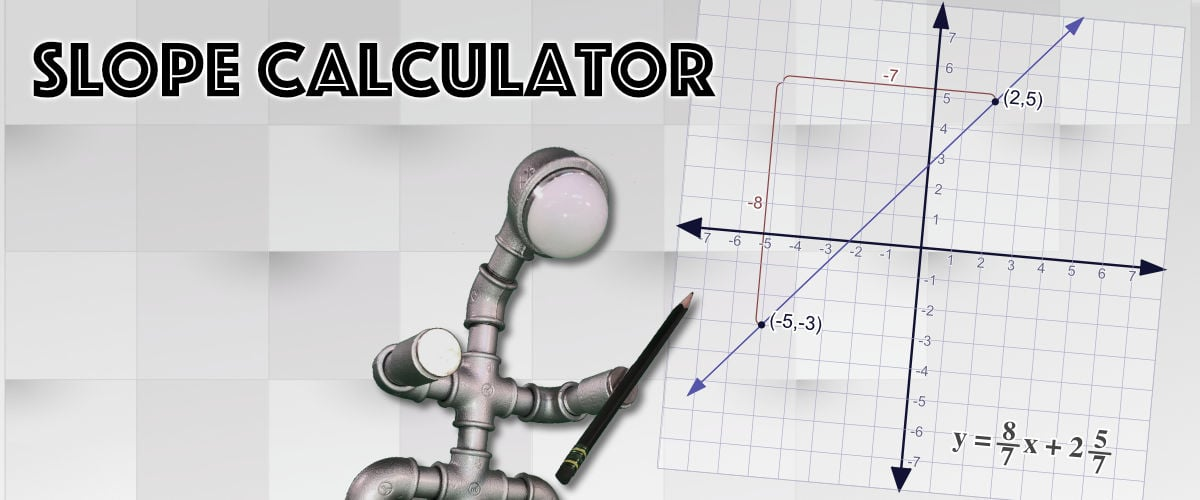One Dad. Four daughters. 9,393 worksheets... and counting!# Calculating the Slope into Summer… It’s All Downhill From Here!

As we slide gently into the final weeks of the school year, a familiar math topic surfaced in our current math curriculum… The slope intercept equation and graphing on the coordinate plane. Remember y = mx + b anyone?

Our 4th grader came home with some work on this topic, I think with more of a goal of exposure in preparation of next year’s rigorous treatment of linear equations, but it did remind me that this was a math topic I had wanted to address on the site for some time.

When you’re learning about slopes and intercepts and graphs and coordinate planes, you wind up drawing lots of pictures (and using lots of graph paper), which can get tedious. Especially if your lazy Dad gets a few of the drawings wrong.

That’s where this graphing slope calculator comes to the rescue…

#### Slope Calculator

This calculator calculates the equation of a line from the two points you enter. It shows how the slope is calculated, including reducing the rise-over-run fraction and giving a decimal equivalent. There’s also a nice summary on that page of how the calculator finds the y-intercept value once the slope and one of the points is known using the recast of the slope intercept equation as b = y – mx.

You can enter the points or the intercept or the slope and the graph will update in real time… This saves a ton of manual graphing to explain concepts, and lets you move quickly between different graphs to explain things like positive versus negative versus zero slope lines. The interactive experience definitely makes explaining these ideas easier, and I’m sure this would be amazing on a classroom smartboard.

I built this calculator using Vue.js, and it’s the first addition to the site using these tools since I built the Worksheet Connect app last year. I’m hoping to use Vue to rebuild some of the other calculators on the site to make them even snazzier, but meanwhile I hope you’ll check out the Connect app to see if it might help you with some summer math fact practice!

Have a great slide into summer! 🙂